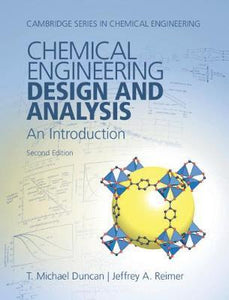# Chemical Engineering Design and Analysis : An Introduction by Duncan, T. Michael

• R 837.75
Unit price per
Tax included.

ISBN: 9781108421478

Cambridge University Press , 31 January 2019

Hardback, 588 pages

Description

This textbook puts design at the center of introducing students to the course in mass and energy balances in chemical engineering. Employers and accreditations increasingly stress the importance of design in the engineering curriculum, and design-driven analysis will motivate students to dig deeply into the key concepts of the field. The second edition has been completely revised and updated. It introduces the central steps in design and three methods of analysis: mathematical modeling, graphical methods, and dimensional analysis. Students learn how to apply engineering skills, such as how to simplify calculations through assumptions and approximations; how to verify calculations, significant figures, spreadsheets, graphing (standard, semi-log and log-log); and how to use data maps, in the contexts of contemporary chemical processes such as the hydrogen economy, petrochemical and biochemical processes, polymers, semiconductors, and pharmaceuticals.